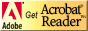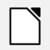Pages created and updated by Terry Sturtevant Date Posted: January 29, 2020

# CP316: Microprocessor Systems and Interfacing

## Pulse Width Modulation

### Objectives

Since creating square waves and pulses is common in circuits, hardware timers make this easy. Once set up, they can run indefinitely without software intervention.

### Equipment

• Arduino Uno
• AVR Data Sheet [pdf,660pp; ©2015]
• oscilloscope
• SG90 servo motor
• Servo motor modified for continuous rotation

### Procedure

In both fast PWM mode and phase corect PWM mode, there are two options for each timer:
1. One where output is on OCxA, the period is determined by MAX, and the duty cycle is determined by OCRxA.
2. One where output is on OCxB, the period is determined by OCRxA, and the duty cycle is determined by OCRxB.
You are going to adapt one sketch to examine each of those 4 options for one timer.

#### Timer features - Fast PWM mode - period determined by MAX

1. Connect the Arduino board.
3. Adapt the sketch for the desired mode.
What changes need to be made to the configuration?
4. Connect the oscilloscope to output pins 3 and 11.
What are those pins in terms of Timer 2?
5. Run the program to see that the square wave appears on the scope.
Sketch the output, and note the oscilloscope settings.
What are the maximum and minimum frequencies available in this mode?
6. Vary the OCRA value to determine how it is related to duty cycle.
Sketch the output, and note the oscilloscope settings.
What happens if the value in OCRB is greater than the value in OCRA? Is that a problem?
Demonstration - demonstrate what you have learned.

#### Timer features - Fast PWM mode - period determined by OCRA

1. Adapt the sketch for the desired mode.
What changes need to be made to the configuration?
2. Connect the oscilloscope to both output pins.
3. Run the program to see the output on the scope.
Sketch the output, and note the oscilloscope settings.
Does the frequency make sense? Explain.
When during the period does the pulse occur?
Do the two different signals make sense? Explain.
4. Vary the OCRA value to determine how it is related to the period.
What are the maximum and minimum frequencies available in this mode?
5. Vary the OCRB value to determine how it is related to duty cycle.
Sketch the output, and note the oscilloscope settings.
What happens if the value in OCRB is greater than the value in OCRA? Is that a problem?
Demonstration - demonstrate what you have learned.

#### Timer features - Phase correct PWM - period determined by MAX

Since the phase correct PWM timer counts up and down, the frequency will be half the frequency given by the corresponding settings in fast PWM mode.
1. Adapt the sketch for the desired mode.
What changes need to be made to the configuration?
2. Connect the oscilloscope to both output pins.
3. Run the program to see the output on the scope.
Sketch the output, and note the oscilloscope settings.
Does the frequency make sense? Explain.
Do the two different signals make sense? Explain.
What are the maximum and minimum frequencies available in this mode?
4. Vary the OCRA value to determine how it is related to duty cycle.
Sketch the output, and note the oscilloscope settings.
What happens if the value in OCRB is greater than the value in OCRA? Is that a problem?
Demonstration - demonstrate what you have learned.

#### Timer features - Phase correct PWM - period determined by OCRA

Since the phase correct PWM timer counts up and down, the frequency will be half the frequency given by the corresponding settings in fast PWM mode.
1. Adapt the sketch for the desired mode.
What changes need to be made to the configuration?
2. Connect the oscilloscope to both output pins.
3. Run the program to see the output on the scope.
Sketch the output, and note the oscilloscope settings.
Does the frequency make sense? Explain.
When during the period does the pulse occur?
Do the two different signals make sense? Explain.
4. Vary the OCRA value to determine how it is related to the period.
What are the maximum and minimum frequencies available in this mode?
5. Vary the OCRB value to determine how it is related to duty cycle.
Sketch the output, and note the oscilloscope settings.
What happens if the value in OCRB is greater than the value in OCRA? Is that a problem?
Demonstration - demonstrate what you have learned.

#### Arduino PWM features - analogWrite() function

1. Create a new sketch, and download the simple analog write sketch.
2. The analogWrite() function uses timers, so the pins that can use it are all timer output pins.

For each of the 3 timers,
1. Identify an output pin associated with that timer.
2. Modify the code to use that output pin.
3. Connect the oscilloscope to that output pin.
4. Determine the frequency and the duty cycle of the output.

Do the timers all work the same way? Explain.
Now assign the output to a pin not associated with any timer. Does it work as you expected? Explain.
3. For one of the timers, vary the BRIGHTNESS value to determine how it is related to duty cycle.
Demonstration - demonstrate what you have determined.

#### Direct PWM servo motor

Servo motors usually use a pulse with a period of 20mS. Which of the timer options above allow that?
1. Open the sketch required for the mode you need.
2. Determine the period parameters needed for a 20ms signal.
3. Determine the duty cycle parameter needed for the neutral position and the two limits.
4. Connect the oscilloscope to the appropriate pin, and see that the waveform is what you expect.
5. Connect the servo motor to the appropriate pin, and see if the motor is in the neutral position. Adjust the code as necessary.
If choosing the "neutral" PWM value doesn't stop the motor, is there another value that would stop the motor for another reason? If so, test and see.
6. Now test both limits, and adjust the code as necessary. Be sure your code doesn't grind the motor against either physical limit.
7. Test the stepsize between one limit and the other. How many steps are there between the two limits?
Demonstration - demonstrate what you have determined.

#### Direct PWM servo motor control for continuous rotation

2. Connect the servo motor to the appropriate pin, and see if the motor is in the neutral position; i.e. stopped. Adjust the code as necessary.
Can you actually choose a value where the motor is stopped?
Was the neutral position in the same place as for the regular servo?
3. Now test both limits, and adjust the code as necessary. Note in this case the limits will be when the motor speed has reached its maximum in either direction.
4. Test the stepsize between one limit and the other. How many steps are there between the two limits?
Demonstration - demonstrate what you have determined.

#### Arduino PWM features - Servo library

2. The servo library uses Timer 1, but is not restricted to Timer 1 output pins.
Verify this, by using output pins that belong to other timers.
For each of the 2 timers, (0 and 2),
1. Identify an output pin associated with that timer.
2. Modify the code to use that output pin.
3. Connect the oscilloscope to that output pin.
4. Determine the frequency and the duty cycle of the output.

Do the pins all work the same way? Explain.
Now assign the output to a pin not associated with any timer. Does it work as you expected? Explain.
3. For one of the pins, vary VAL to determine how it is related to duty cycle.
Demonstration - demonstrate what you have determined.

#### Servo library for servo motor control

1. Now use the servo library code with the normal (SG90) servo motor.
2. Connect the servo motor to an appropriate pin, and see if the motor is in the neutral position. Adjust the code as necessary.
3. Determine the duty cycle parameter needed for the neutral position and the two limits.
How does this compare to using the timers directly?
Demonstration - demonstrate what you have determined.

#### Servo library for continuous rotation servo motor control

1. Connect the continuous rotation servo motor to the appropriate pin, and see if the motor is in the neutral position; i.e. stopped. Adjust the code as necessary.
Can you actually choose a value where the motor is stopped?
Was the neutral position in the same place as for the regular servo?
How do these compare to controlling the timers directly?
2. Now test both limits, and adjust the code as necessary. Note in this case the limits will be when the motor speed has reached its maximum in either direction.
Demonstration - demonstrate what you have determined.
3. #### PWM and H-Bridge for DC motor control## ResourcesIf you need to update a browser, you might try Firefox which is
• free
• open source
• available for several platformsSince this page uses cascading style sheets for its layout, it will look best with a browser which supports the specifications as fully as possible.

If you are looking for an office package, with a word processor, spreadsheet, etc., you might try LibreOffice which is
• free
• open source
• available for several platformsGo to the main page for the Department of Physics and Computer Science.

Wilfrid Laurier University